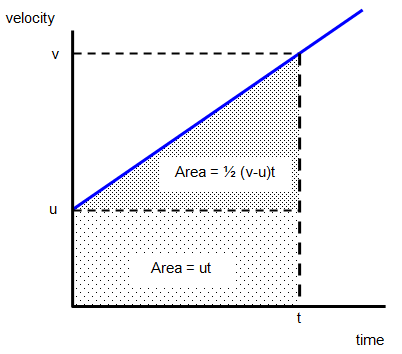# Equations of motion proof

In studying the motion of objects it is often helpful to use an equation to work out the velocity, acceleration or the distance travelled.

We use the following letters to represent certain quantities:

 Distance travelled s measured in metres (m) Time taken t measured in seconds (s) Initial velocity u measured in metres per second (ms-1) Final velocity v measured in metres per second (ms-1) Acceleration a measured in metres per second per second (ms-2)

## 1. Non accelerated motion – that is motion at a constant velocityThe area under the line of the velocity–time graph is the distance travelled by the object in the time t.

For example u = 20m/s and t = 300 s Distance (s) = ut = 20 x 300 = 6000 m

The equation for non accelerated motion is:

Distance (s) = velocity (u or v) x time (t)
s = vt

## 2. Accelerated motion

Acceleration (a) = [change in velocity]/time = [v - u]/t
or
a = [v - u]/t

Another version is:

v = u + atDistance travelled = area under the line = ut + ˝ (v-u)t

But acceleration = (v-u)/t and so (v-u) = at therefore:

Distance travelled (s) = ut + ˝ (v-u)t = ut + ˝ [at]t = ut + ˝ at2

s = ut + ˝ at2

If the object starts from rest u = 0 and so the equation becomes:

s = ˝ at2

Another useful equation is:

v2 = u2 + 2as

This equation can be proved as follows:
v = u + at therefore t = (v-u)/a but s = ut + ˝ at2 and so

s = ut + ˝ a([v-u]/a)2 therefore: 2s = 2u(v-u)/a + (v2 – 2uv + u2)/a

So: 2as = 2uv – 2u2 + v2 – 2uv + u2 and so v2 = u2 + 2as

Equations summary

CONSTANT VELOCITY
1. s = vt

CONSTANT ACCELERATION
2. v = u + at
3. s = ut + ˝ at2
4. average velocity = [v + u]/t
5. v2 = u2 + 2as

## USING EQUATIONS

This section is designed to help you work out some of the problems using the equations of motion.

Example problems
1. A horse accelerates steadily from rest at 4 ms-2 for 3s.
What is its final velocity and how far has it travelled?
(a) Initial velocity (u) = 0 Final velocity (v) = ?
Acceleration (a) = 4 ms-2 Time (t) = 3 s.
Known quantities u,a and t. Unknown v.
Correct equation is v = u + at
Final velocity (v) = 0 + 4x3 = 12 ms-1
(b) Correct equation is s = ut + ˝ at2
Distance (s) = 0x3 + 0.5x4x9 = 18 m

2. A car travelling at 20 ms-1 accelerates steadily at 5 ms-2 for a distance of 70 m. What is the final velocity of the car?
Known quantities u,a and s. Unknown v.
Correct equation is v2 = u2 + 2as
Final velocity2 (v2) = 30x30 + 2x5x70
Therefore v = 40 ms-1

If you need to use any of these equations to work out problems the way to do it is this:

(a) write down what you are given, usually three things
(b) look for the equation that contains these three things and the quantity that you are trying to find
(c) put the numbers in the CORRECT equation and work it out

You will need to know how to rearrange equations to make different quantities the subject of the equation.

A VERSION IN WORD IS AVAILABLE ON THE SCHOOLPHYSICS USB

© Keith Gibbs 2020